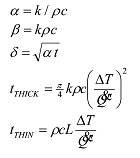# Ignition of thermal insulator

#### Eva Brain

1. The problem statement, all variables and given/known data

A new type of insulating board has been developed by that esteemed construction company Kaput Ltd. They warn that at extremely high heat fluxes it could be ignited, but they don’t think it’s very likely and it would take hours, so there’s no real risk!

As an expert on the ignitability of materials you are asked to perform a thick/thin calculation given the following data on the material:

Density 2400 kg m-3
Thermal conductivity 0.85 W m-1 K-1
Specific heat capacity 827 J kg-1 K-1
Thickness of board 7mm
Initial/ambient laboratory temperature 18°C
Ignition temperature 410°C
It is considered that if 20 kW m-2 would be enough to ignite most materials (i.e. indicative of flashover fires). Perhaps Something easy to ignite would only require 10 kW m-2. Would this material ignite within ten minutes if exposed to 10 kW m-2?

2. Relevant equations3. The attempt at a solution

Don't know how to start this.

#### mfb

Mentor
You could identify the given numbers with the symbols in your formulas and plug in all known values there afterwards. What can you calculate, what is missing?

#### Eva Brain

You could identify the given numbers with the symbols in your formulas and plug in all known values there afterwards. What can you calculate, what is missing?
What value go with what symbol?

#### SteamKing

Staff Emeritus
Homework Helper
What value go with what symbol?
Don't you have a textbook or some class notes? Where did you get the formulas shown in the OP?

#### Eva Brain

Don't you have a textbook or some class notes? Where did you get the formulas shown in the OP?
Yes, but in textbooks symbols are all different! Can you show me what is what symbol, I will try to solve it?

Last edited:

#### SteamKing

Staff Emeritus
Homework Helper
Yes, but in textbooks symbols are all different! Can you show me what is what symbol, I will try to solve it?
You still aren't answering the important question: If you didn't get these formulas from your textbook, where did they come from?

#### Eva Brain

You still aren't answering the important question: If you didn't get these formulas from your textbook, where did they come from?
From a task. It was included in task. But in my textbooks it is completely different symbols.

#### Eva Brain

OK, I have done following:

Density 2400 kg m-3 ( p )
Thermal conductivity 0.85 W m-1 K-1 ( k )
Specific heat capacity 827 J kg-1 K-1 ( c )
Thickness of board 7mm ( t )
Initial/ambient laboratory temperature 18°C (T_0 )
Ignition temperature 410°C ( T_ig )

Found alpha, beta and delta.
Alpha = 0.003
Beta = 1687080
Delta = 0.0045

q_critical is given as 20 000 W / m^-2

Delta T = (T_ig - T_0) / q_critical.

Problem is finding value of L. I don't know if there is general formula for that, but I deem based on given delta we can find out something. Nonetheless, I require your help since I am so close to close even this one.

#### Eva Brain

OK, I have done following:

Density 2400 kg m-3 ( p )
Thermal conductivity 0.85 W m-1 K-1 ( k )
Specific heat capacity 827 J kg-1 K-1 ( c )
Thickness of board 7mm ( t )
Initial/ambient laboratory temperature 18°C (T_0 )
Ignition temperature 410°C ( T_ig )

Found alpha, beta and delta.
Alpha = 0.003
Beta = 1687080
Delta = 0.0045

q_critical is given as 20 000 W / m^-2

Delta T = (T_ig - T_0) / q_critical.

Problem is finding value of L. I don't know if there is general formula for that, but I deem based on given delta we can find out something. Nonetheless, I require your help since I am so close to close even this one.

### The Physics Forums Way

We Value Quality
• Topics based on mainstream science
• Proper English grammar and spelling
We Value Civility
• Positive and compassionate attitudes
• Patience while debating
We Value Productivity
• Disciplined to remain on-topic
• Recognition of own weaknesses
• Solo and co-op problem solving